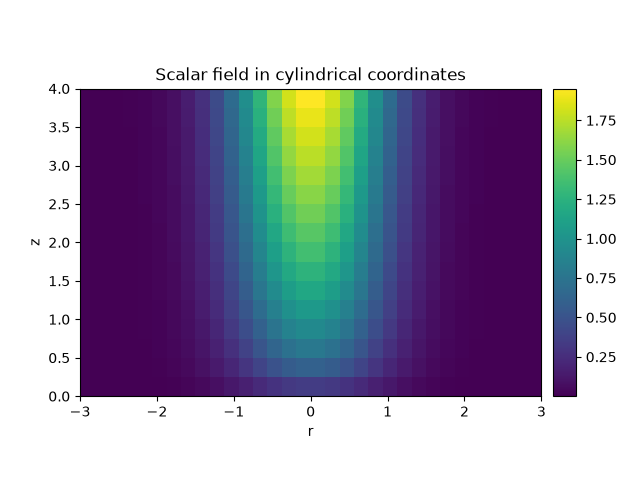# 2.3. Plotting a scalar field in cylindrical coordinates

This example shows how to initialize and visualize the scalar field $$u = \sqrt{z} \, \exp(-r^2)$$ in cylindrical coordinates.from pde import CylindricalSymGrid, ScalarField

grid = CylindricalSymGrid(radius=3, bounds_z=[0, 4], shape=16)
field = ScalarField.from_expression(grid, "sqrt(z) * exp(-r**2)")
field.plot(title="Scalar field in cylindrical coordinates")


Total running time of the script: (0 minutes 0.469 seconds)